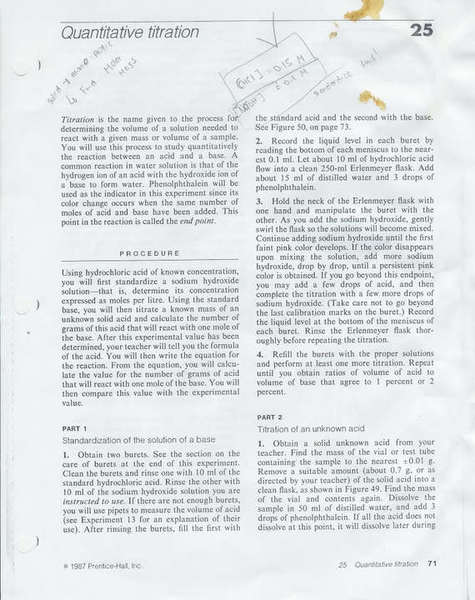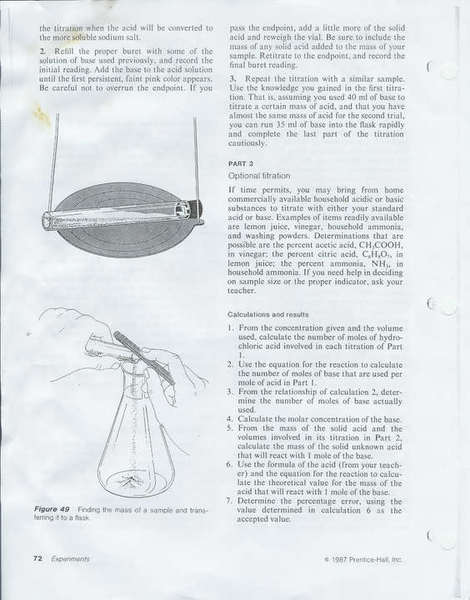Quantitative Titration - NaOH and HCl

Homework Statement

Hi, So I have this lab in which we had to titrate HCl with NaOH (of an unknown molarity)So here's the lab.. I decided to scan it because I'm not a good explainer at all, heh.
The molarity of the HCl is 0.15M by the way

What I was wondering is how to calculate #2 under calculations and results... For our titration of the HCl, we had gotten 15.8mL (average) of NaOH used. For calculations, however, I used the concentration of the diluted acid (0.06M for the 25mL of water and HCl combined.. which I dont know whether it's right or not) to calculate that the molarity of the NaOH is 0.095M

Homework Equations

CaVa=CbVb

And would we have to use Kw = [H+][OH-] in any way here?

The Attempt at a Solution

CaVa=CbVb
(0.06M)(0.025L) = (Mb)(0.0158)L)
Mb=0.095M

For question 2 it refers to the chemical equation which is:
HCl + NaOH -> NaCL + H2O

In which case the moles of base used per mole of acid is 1. It is a 1:1 ration, and your titration shows this.
M(base) = n/V(L)
.095(.0158) = n
n = .0015
and
M(acid) = n/V(L)
.06(.025) = n
n= .0015

a 1:1 mole ratio

Borek
Mentor
however, I used the concentration of the diluted acid (0.06M for the 25mL of water and HCl combined.. which I dont know whether it's right or not)

You don't know exact volume (about+about is not exactly 25) so you can't calculate exact concentration of HCl. However, it is all about number of moles of acid - did it changed after dilution?

You may consult www.titrations.info - a lot of information about titrations, acid/base ones included.

For question 2 it refers to the chemical equation which is:
HCl + NaOH -> NaCL + H2O

In which case the moles of base used per mole of acid is 1. It is a 1:1 ration, and your titration shows this.
M(base) = n/V(L)
.095(.0158) = n
n = .0015
and
M(acid) = n/V(L)
.06(.025) = n
n= .0015

a 1:1 mole ratio

Oh really? Haha, I thought it was asking for something more difficult! Thank youYou don't know exact volume (about+about is not exactly 25) so you can't calculate exact concentration of HCl. However, it is all about number of moles of acid - did it changed after dilution?

You may consult www.titrations.info - a lot of information about titrations, acid/base ones included.

Wait, since it's not accurate enough, then I assume that I can't follow through with Nickdk's solution then?

Borek
Mentor
What he calculated using numbers you gave is acidentally correct, but it is not how it should be done.

You should calculate number of moles of the acid introduced to the solution - that means precisely measured 10 mL of 0.15M solution, or 1.5 mmole.

Now, after solution was diluted to ABOUT 25 mL, its concentration went down to ABOUT 0.06M. However, new concentration is calculated assuming number of moles of acid have not changed - and you need this number of moles to calculate number of moles of base that reacted. And you have already calculated that it was 1.5 mmole.

If so, do you need to calculate new concentration after dilution? You divide number of moles by approximate volume and then you multiply it again by the same approximate value - so approximate volume cancels out and fact that it was an approximate value doesn't matter any longer, question is - was this operation necessary?

Last edited by a moderator:
What he calculated using numbers you gave is acidentally correct, but it is not how it should be done.

You should calculate number of moles of the acid introduced to the solution - that means precisely measured 10 mL of 0.15M solution, or 1.5 mmole.

Now, after solution was diluted to ABOUT 25 mL, its concentration went down to ABOUT 0.06M. However, new concentration is calculated assuming number of moles of acid have not changed - and you need this number of moles to calculate number of moles of base that reacted. And you have already calculated that it was 1.5 mmole.

If so, do you need to calculate new concentration after dilution? You divide number of moles by approximate volume and then you multiply it again by the same approximate value - so approximate volume cancels out and fact that it was an approximate value doesn't matter any longer, question is - was this operation necessary?

--

Hi, I'm back again, sorry to be such a bother.. But I was wondering if it were possible to find the molar mass of the unknown salt (as our teacher instructed us to do) just from the given information. Since there's no concentration of the solid, how would it be possible to find the molar mass of it? Would I have to use the concentration of the acid dissolved in the water as the conentration to solve? And if I were to use the concentration of that solution, how would I go about doing that? Any help on this will be appreciatedBorek
Mentor
Not sure what you are asking about (no idea what your insctructor told you and what information you can use), but usually these things are done using known mass of the solid acid and determining number of moles of the acid by titration - if you know mass of the sample and number of moles, finding molar mass is a breeze.

Last edited by a moderator:
Not sure what you are asking about (no idea what your insctructor told you and what information you can use), but usually these things are done using known mass of the solid acid and determining number of moles of the acid by titration - if you know mass of the sample and number of moles, finding molar mass is a breeze.

--

Well, this is what I have so far :

mass of acid = 0.7g
average volume used for titration= 0.0295L

nNaOH= cv
=(0.095M)(0.0295L) [ the 0.095M is calculated by the way]
=2.8x10-3 mol of base reacting with 0.7g of acid

After this.. I used a ratio to solve for the mass of acid that would react with 1 mol of base ( as #6 called for)

which is about 250g of acid / 1 mol of NaOH

So, after a bit of research, I'm assuming I do no. moles = mass / molar mass..

but the thing is, I don't have the number of moles of acid that I need for the equation..Well that is unless I use the number of moles that reacted with the 0.7 g of acid, then do...

2.8x10-3 mol = 0.7g / Molar mass
Molar mass = 250g/mol

However, this seems a bit too high to belong to any of the strong acids... unless it isn't a strong acid maybe?

Borek
Mentor
However, this seems a bit too high to belong to any of the strong acids... unless it isn't a strong acid maybe?

It can be some other acid as well. And 250 is a correct result (that is, assuming numbers you have listed are correct).

Just note that this is not a molar mass, but so called equivalent mass - you don't know if this is mono- or multiprotic acid.

Last edited by a moderator: